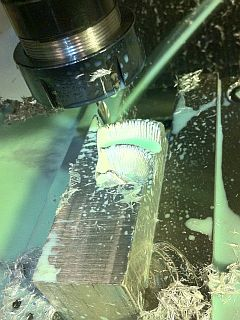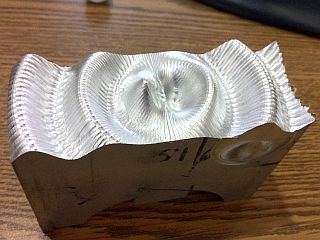# CNC Function Mill

## Overview

This project was a quick proof of concept for using a CNC with a ball-end mill to create 3D profiles based on an input function. The function used here represents the field around an electric dipole oscillator. A MATLAB script is used to generate a g-code file that is sent to a CNC mill. First the script generates the values of the function on a cylindrical grid (radius and angle from central point, height from base plane). It is inadequate to simply give these function outputs as 3D coordinate for the mill, as this will destroy the 3D slope data by forcing the slope to be zero (this is the slope at the bottom of the ball end mill) at every point and whatever corresponds to the end mill radius at every surrounding point. Instead the program finds the function's 2D-slope and its normal unit vector at each of the evaluated points and uses that to determine the 3D location that would correspond to the center of a sphere rolling along the function's curve at that point. It is not too difficult to imagine that the path of the 'ball center' of the ball end mill will need to correspond to these locations in order to mill out the desired function. The maximum negative curvature is still limited to the diameter of the end mill, and without further analysis of the function, which would be a computationally intensive 'collision detection', exceeding this curvature in the function will make points surrounding these areas be milled in an invalid way. The function shown here was tailored to not exceed the required curvature while also being scaled so that the end mill used (0.25 inch diameter, 2 inch length) would be used to its full capacity. The program mills the profile in the radial direction, milling from the center outwards, then shifting to the next angle theta, then milling back inwards to the center and continuing to the next angle. The resulting piece has visible rectangular (actually polar rectangles, so slightly curved) artifacts that show the limited resolution of the calculated points (the CNC mill uses linear extrapolation between points leading to the rectangular sections). Overall the concept worked quite successfully.On left, the aluminum piece is in the CNC mill about 40% through the operation. On right, the finished piece. The width is 2 inches, and length is approximately 6 inches.